The Mathematical City
-- optimized for minimum travel times --
by Egbert Gramsbergen

What is the problem?
Suppose we lay down a big city of skyscrapers on a rectangular grid of streets. We can travel along the streets with a certain velocity v and vertically in the buildings with velocity w. The velocities are constants and there are no waiting times and traffic jams. As you see, a highly realistic model of a real city. Each 3D point in the city (i.e. each floor in each building) attracts random traffic from each other point. What shape should the city have, given fixed volume, in order to minimize the mean travelling time for all journeys? Or, to state it differently, to minimize the total amount of traffic?

The mathematics (or skip the mathematics)
First, let us suppose that v=w. It makes the equations a bit simpler and we can scale the height of the buildings proportionally afterwards. Furthermore, let us assume that the city is really big, so that we can ignore both the grid size of the street pattern (there are many streets) and the hight of the floors (buildings have many floors). We consider the city as a body with a distance between points (x,y,z) and (x',y',z') defined as d=|x-x'|+|y-y'|+z+z'. Within the city z>0, z=0 outside it.
Given a total volume, the integral of all distances from any point P to any other point P' within the body is minimized if the integral of distances from a fixed point Ps on the surface of the body to any other point P' within the body is the same for all Ps (Think about that! By the same token, the sea is flat to minimize its potential energy). So,
 (1)where z(x,y) is the upper surface of the body A is defined by
 (2)Without loss of generality we can center the city around x=y=0 so, anticipating that the solution will have the same symmetry properties as the distance definition, we have the symmetry relations
 (3)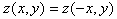(4)which we will use repeatedly. z' can be integrated out so that
 (5)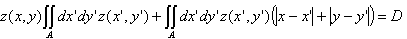where D is a new constant. Differentiating with respect to x and y yields
 (6)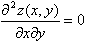so that
 (7)where the functions f1 and f2 can be taken equal by virtue of (4), so
 (8)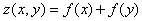Substituting with (8) in (5) with y=0 we obtain
 (9)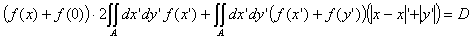where symmetry and interchange of x' and y' were used resulting in the factor 2 in the first term. Defining
 (10)and using symmetry we get
 (11)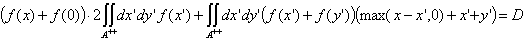or
 (12)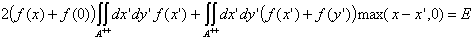with a new constant E. Differentiating to x:
 (13)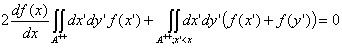We now introduce g(x) as the border of the city:
 (14)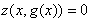or, with (8),
 (15)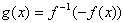and use
 (16)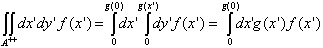and similarly
 (17)and, interchanging x' and y',
 (18)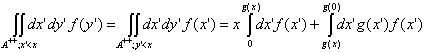(draw a picture for the last step!) to arrive at
 (19)Equations (8), (15) and (19) together determine the shape of the city.
How to proceed from here? In order to get a practical numerical recipe, we take the x-derivative of (19) twice, defining A as
 (20)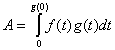and using g(g(x))=x, f(g(x)=-f(x) and general relations like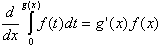with the result
 (21)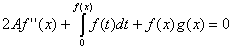and
 (22)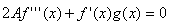or, writing down f'''(x) explicitely:
 (23)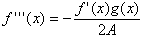The numerical recipe now goes as follows:

2. Calculate g(x) by solving f(x)+f(g(x))=0, which, after the first iteration, must be done numerically. This is a simple task for any modern spreadsheet program. In the steps ahead, we will need to know g(x) for any x, so it is convenient to develop a polynomial expansion or apply some other interpolation scheme.
3. Calculate A with (20)
4. With the now known A, f' and g calculate f'''(x) with (23) for a large number of points x between 0 and g(0). This is the first step of a new iteration cycle with an improved funcion f.
5. Integrate twice, numerically, starting at x=0. This involves two integration constants given byand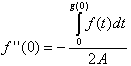. We can take an arbitrary f(0); scaling of the whole city can be done afterwards.
6. Go back to step 2 until f becomes stable in the iteration process.

The result

 f(x)g(x)(quarter circle drawn for comparison)
and the city looks like: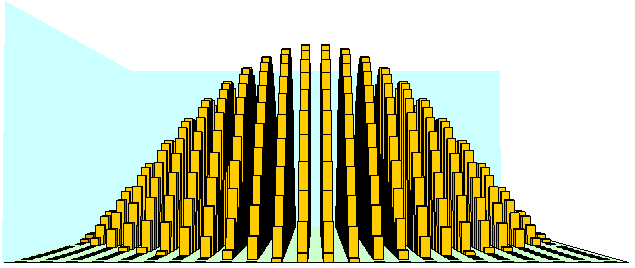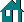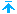created: 1997-08-09last update: 2001-02-20